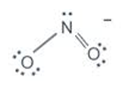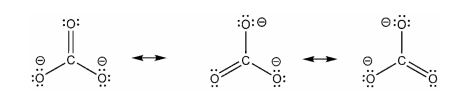# Calculate formal charges on Nitrogen Dioxide (NO2-) anion

Calculate formal charges on Nitrogen Dioxide (NO2-) anion,carbonate anion(CO32-), Carbonate chloride(COCL2)

The charge assigned to an atom in a molecule is formal charge.
Formal charge (FC) is given by the formula,
FC=V-N-B/2

Where,
V= Number of valence electrons
N= Number of non bonding electrons
B= Total number of electrons shared in covalent bonds.The formal charge of N in NO2- is
FC = 5 - 2 - 6/2
=0
For the LHS oxygen the FC is-
FC = 6 - 6 - 2/2
= -1
For the RHS oxygen the FC is-
FC = 6 - 4 - 4/2 = 0
The carbonate ion [CO3 2-] has an overall charge of -2. In the carbonate ion, the carbon atom is bonded with a double bond to an oxygen atom, and with single bonds to two oxygen atoms.he formal charge on each of the atoms can be calculated as follows
Formal charge (FC) is given by the formula
FC=V-N-B/2

Where,
V= Number of valence electrons
N= Number of non bonding electrons
B= Total number of electrons shared in covalent bonds.

FC of carbon = 4 - 0 - 1/2 (4) = 0
Formal charge on oxygen bonded with the double bond = 6 - 4 - 1/2 (4) = 0
Formal charge on oxygen atoms bonded with single bond = 6 - 6 - 1/2 (2) = -1
Similarly you can calculate the formal charge of Carbonate chloride (COCl2).